PEAK-Rules/Predicates UserPreferences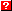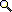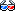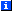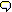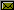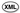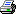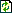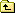The following 739 words could not be found in the dictionary of 50 words (including 50 LocalSpellingWords) and are highlighted below:
Add   Also   And   Any   Arbitrary   Arg   Argument   Arguments   As   At   Attribute   Bar   Binding   Builder   Building   But   By   Center   Class   Code   Compare   Comparison   Compiling   Complex   Conjunction   Const   Contents   Conversion   Criteria   Criterion   Custom   Defining   Dev   Disjunction   Dispatch   Dispatching   Duplicate   Dynamic   Each   Else   Engine   Error   Errors   Except   Expansion   Expr   Expression   False   For   Function   Functions   Future   Generation   Generator   Got   Here   Identity   If   Implemented   In   Independence   Indexed   Indexing   Instance   Is   It   Iterable   Just   Key   L1   L2   L3   L4   L5   L6   Local   Matching   Max   Meta   Method   Min   Missing   Most   Mul   Name   New   None   Normally   Not   Note   Notice   Object   Of   Okay   On   Or   Order   Ordering   Parsing   Pattern   Predicate   Python   Range   Return   Rule   Rules   Scope   Set   Signature   So   Specifically   Static   Subclass   Syntax   Table   Test   That   The   Then   There   This   To   Too   Traceback   True   Truth   Type   Types   Ultimate   Upgrading   Value   You   ability   above   abstract   accept   accidentally   accomplish   according   active   actual   actually   add   affect   affects   after   all   allow   allowed   allows   already   also   although   always   among   an   and   another   answer   any   applied   appropriate   appropriately   arbitrary   are   aren   arg   args   argument   arguments   around   as   assembler   assembles   associated   at   attached   away   backquotes   base   basic   basis   be   because   becomes   been   before   begin   beginning   behavior   being   below   between   bind   binding   bindings   bit   body   boolean   bound   build   builder   building   built   builtins   but   by   bytecode   cache   calculations   call   callable   called   caller   calling   calls   can   cannot   capable   cases   check   class   classes   clause   clauses   closure   code   codegen   com   common   compile   compiled   compiling   complete   completed   computed   condition   conditions   confirm   constant   constants   constraints   contain   contents   context   conversion   conversions   converted   converts   copy   core   correct   corresponding   course   create   created   creating   criteria   custom   data   decorate   decorator   def   default   define   defined   defining   definition   demo   dependent   depending   details   detected   determination   dict   dictionary   didn   dis   disjunctive   disjuncts   dispatch   dispatching   do   document   documents   does   don   done   double   dstar   dummy   dummy2   dump   duplicate   dynamic   each   easier   ed   effect   effects   efficiency   efficient   either   element   empty   encountered   engine   engines   entry   equality   errors   escape   etc   evaluated   even   event   every   exact   example   except   exception   executed   execution   existing   expand   expanded   expansion   expects   expr   expression   expressions   exprs   extra   extremes   fact   failure   false   feature   first   five   flags   for   form   found   from   frozenset   full   function   functions   general   generated   generating   generic   get   gets   getting   given   globals   had   handle   handled   handles   happens   has   have   having   heck   helper   how   however   id   idea   identical   identity   if   illustrate   immediate   implement   implementation   implication   implies   import   imported   improve   in   include   independent   indexing   indicates   influence   initialized   inside   inspection   instance   instead   int   integer   interface   interfere   internals   interpret   into   invocation   invoke   invokes   involved   is   isinstance   isint   isisntance   isn   issubclass   istype   it   iterable   its   itself   just   k1   k2   keeps   keyword   keywords   kind   kw   lambda   last   left   let   lets   letter   level   like   list   ll   local   locals   locate   logical   long   looked   looking   looks   lookup   lower   macros   main   make   manipulate   manipulation   manual   many   match   matched   matching   means   memo   meta   method   methods   might   module   moment   more   most   must   name   named   names   necessary   need   needed   needs   negations   neither   never   new   next   no   node   nodes   nodetype   non   normal   not   note   nothing   number   ob   object   objects   obtains   occasion   occur   oddment   of   ok   old   on   once   one   only   operation   operator   operators   opposed   optional   or   order   ordering   original   other   otherwise   our   out   over   overall   overflow   overkill   own   p1   p2   packed   pair   parameter   parameters   parse   parsed   parsing   part   pass   passed   passing   pasting   pattern   patterns   pe   peak   perhaps   place   plain   position   positional   possible   precedence   precomputed   predicate   predicates   previous   print   priorities   priority   problems   process   produces   prove   provided   pseudo   raise   range   ranges   raw   recalculated   recalculating   received   recent   recursively   redefined   redo   reduced   refer   referenced   regardless   register   registered   registering   remaining   rendered   replace   representation   representing   reserved   restored   restrictions   resulting   return   returns   retyping   right   rule   rules   runtime   s1   s2   safely   said   same   saves   say   second   section   see   seen   select   selected   selection   selects   should   side   signature   signatures   simple   simplified   simply   since   so   some   somepriority   something   somewhat   sort   source   special   specialized   specify   split   stack   standard   star   static   still   str   string   structure   stub   sub   subclass   subject   subnode   such   supplied   supply   support   supporting   supposed   sure   surrounding   sync   syntax   takes   target   telecommunity   temporary   test   testable   tested   tests   text   than   that   the   their   them   then   there   these   they   thing   things   this   though   time   tmp   to   transform   transformations   treated   tree   tricky   trivial   true   truth   try   trying   tuple   tuples   type   types   unicode   unpacks   until   up   upgrade   upgrading   usable   use   used   uses   using   util   value   values   variable   variables   ve   versions   via   virtually   want   wants   was   way   we   were   what   whatever   when   whenever   where   whether   which   whose   will   wish   with   within   won   work   works   would   wrap   wrapper   write   wxyz   xx   yeah   you   your   zero

# Predicate Dispatch

Here, we document and test the internals of PEAK-Rules' predicate dispatch implementation. Specifically, how it assembles the things we've already seen in the AST Building, Code Generation, Criteria and Indexing documents, into a complete implementation.

# Predicate Expression Types

Predicate expression types wrap expressions to specify what kind of dispatching should be done on the base expression. For example, predicates.IsInstance indicates that an expression is to be looked up by what it's an instance of.

There are five built-in expression types:

```>>> from peak.rules.predicates import \
...     Truth, Identity, Comparison, IsSubclass, IsInstance
```

And we will test them using code objects:

```>>> from peak.util.assembler import Code, Const, dump
>>> from dis import dis
```

## Truth

The Truth predicate tests whether its subject expression is true or false, and selects the appropriate sub-node from a (true_node, false_node) tuple:

```>>> c = Code()
>>> c(Truth(42))
>>> dump(c.code())
LOAD_FAST                0 (\$Arg)
UNPACK_SEQUENCE          2
LOAD_CONST               1 (42)
JUMP_IF_TRUE            L1
ROT_THREE
L1:     POP_TOP
ROT_TWO
POP_TOP
```

The generated code unpacks the 2-tuple, and then does a bit of stack manipulation to select the correct subnode.

The disjuncts() of a Truth Test is the Test itself:

```>>> from peak.rules.core import disjuncts
>>> from peak.rules.criteria import Test, Signature, Value

>>> disjuncts(Test(Truth(88), Value(True)))
[Test(Truth(88), Value(True, True))]

>>> disjuncts(Test(Truth(88), Value(True, False)))
[Test(Truth(88), Value(True, False))]
```

## Identity

The Identity predicate looks up the id() of its subject expression in a dictionary of sub-nodes. If the id isn't found, the None entry is used:

```>>> c = Code()
>>> c(Identity(99))
>>> dump(c.code())
LOAD_CONST               1 (<built-in function id>)
LOAD_CONST               2 (99)
CALL_FUNCTION            1
DUP_TOP
LOAD_FAST                0 (\$Arg)
COMPARE_OP               6 (in)
JUMP_IF_FALSE           L1
POP_TOP
LOAD_FAST                0 (\$Arg)
ROT_TWO
BINARY_SUBSCR
JUMP_FORWARD            L2
L1:     POP_TOP
POP_TOP
LOAD_FAST                0 (\$Arg)
LOAD_CONST               0 (None)
BINARY_SUBSCR
```

## Comparison

The Comparison predicate expects its "arg" to be an (exact, ranges) pair, such as might be generated by the peak.rules.indexing.split_ranges function:

```>>> c = Code()
>>> c(Comparison(555))
>>> dis(c.code())
0           0 LOAD_CONST               1 (<function value_check at ...>)
3 LOAD_CONST               2 (555)
6 LOAD_FAST                0 (\$Arg)
9 CALL_FUNCTION            2
```

The generated code simply calls a helper function, value_check, with its expression and argument. The helper function looks up and returns the appropriate subnode, first by trying for an exact match, and then looking for a range match if no exact match is found:

```>>> from peak.rules.predicates import value_check
>>> from peak.util.extremes import Min, Max

>>> exact = {'x':1, 'y':2}
>>> ranges = [((Min,'x'),42), (('x','y'),99), (('y',Max),88)]

>>> for letter in 'wxyz':
...     print value_check(letter, (exact, ranges))
42
1
2
88
>>> value_check('xx', (exact, ranges))
99
```

## IsSubclass

The IsSubclass predicate uses a (cache, lookup) node pair, where cache is a dictionary from classes to nodes, and lookup is a function to call with the class, in the event that the target class isn't found in the cache:

```>>> c = Code()
>>> c(IsSubclass(Const(int)))
>>> dump(c.code())
LOAD_CONST               1 (<type 'int'>)
SETUP_EXCEPT            L1
DUP_TOP
LOAD_FAST                0 (\$Arg)
UNPACK_SEQUENCE          2
ROT_THREE
POP_TOP
BINARY_SUBSCR
ROT_TWO
POP_TOP
POP_BLOCK
JUMP_FORWARD            L3
L1:     DUP_TOP
LOAD_CONST               2 (<...KeyError...>)
COMPARE_OP              10 (exception match)
JUMP_IF_FALSE           L2
POP_TOP
POP_TOP
POP_TOP
POP_TOP
LOAD_FAST                0 (\$Arg)
UNPACK_SEQUENCE          2
POP_TOP
ROT_TWO
CALL_FUNCTION            1
JUMP_FORWARD            L3
L2:     POP_TOP
END_FINALLY
```

## IsInstance

The IsInstance predicate is virtually identical to IsSubclass, except that it first obtains the __class__ or type() of its target:

```>>> c = Code()
>>> c(IsInstance(Const(999)))
>>> dump(c.code())
LOAD_CONST               1 (999)
SETUP_EXCEPT            L1
DUP_TOP
LOAD_ATTR                0 (__class__)
ROT_TWO
POP_TOP
POP_BLOCK
JUMP_FORWARD            L3
L1:     DUP_TOP
LOAD_CONST               2 (<...AttributeError...>)
COMPARE_OP              10 (exception match)
JUMP_IF_FALSE           L2
POP_TOP
POP_TOP
POP_TOP
POP_TOP
LOAD_CONST               3 (<type 'type'>)
ROT_TWO
CALL_FUNCTION            1
JUMP_FORWARD            L3
L2:     POP_TOP
END_FINALLY
L3:     SETUP_EXCEPT            L4
DUP_TOP
LOAD_FAST                0 (\$Arg)
UNPACK_SEQUENCE          2
ROT_THREE
POP_TOP
BINARY_SUBSCR
ROT_TWO
POP_TOP
POP_BLOCK
JUMP_FORWARD            L6
L4:     DUP_TOP
LOAD_CONST               4 (<...KeyError...>)
COMPARE_OP              10 (exception match)
JUMP_IF_FALSE           L5
POP_TOP
POP_TOP
POP_TOP
POP_TOP
LOAD_FAST                0 (\$Arg)
UNPACK_SEQUENCE          2
POP_TOP
ROT_TWO
CALL_FUNCTION            1
JUMP_FORWARD            L6
L5:     POP_TOP
END_FINALLY
```

## Defining New Predicate Types

A predicate type must be a peak.util.assembler.nodetype, capable of generating its own lookup code. The code will be used in a SMIGenerator context (see the Code Generation manual), so SMIGenerator.ARG will contain a lookup node.

Each predicate type must be usable with the predicates.predicate_node_for function, and the predicates.always_testable function:

predicate_node_for(builder, expr, cases, remaining_exprs, memo)
Return a dispatch tree node argument appropriate for the expr. The return value(s) of this function will be in the SMIGenerator.ARG local variable when the predicate type's bytecode is executed.
always_testable(expr)
Return true if the expression can always be tested, regardless of its position among the signature condition(s). Most predicate types should just implement this by calling always_testable() recursively on their target expression, and in fact all of the built-in predicate types do this. For more details, see the section on Order Independence below.

# Predicate Parsing

The CriteriaBuilder class can be used to parse Python expressions into tests and signatures. It's initialized using the same arguments as the codegen.ExprBuilder class:

```>>> from peak.rules.predicates import CriteriaBuilder, Comparison, istype
>>> from peak.rules.criteria import Disjunction, Value, Test, Range, Class, OrElse
>>> from peak.util.assembler import Local

>>> builder = CriteriaBuilder(
...     dict(x=Local('x'), y=Local('y')), locals(), globals(), __builtins__
... )
>>> pe = builder.parse

>>> pe('x+42 > 23*2')
Test(Comparison(Add(Local('x'), Const(42))), Range((46, 1), (Max, 1)))
```

The in operator converts constant classes and istype() expressions into IsInstance tests:

```>>> pe('x in int')
Test(IsInstance(Local('x')), Class(<type 'int'>, True))

>>> pe('x not in int')
Test(IsInstance(Local('x')), Class(<type 'int'>, False))

>>> pe('x in istype(int)')
Test(IsInstance(Local('x')), istype(<type 'int'>, True))

>>> pe('x not in istype(int)')
Test(IsInstance(Local('x')), istype(<type 'int'>, False))

>>> pe('x in istype(int, False)')
Test(IsInstance(Local('x')), istype(<type 'int'>, False))

>>> pe('x not in istype(int, False)')
Test(IsInstance(Local('x')), istype(<type 'int'>, True))
```

Iterable constants into or-ed equality tests:

```>>> pe('x in (1,2,3)') == Disjunction([
...     Test(Comparison(Local('x')), Value(2, True)),
...     Test(Comparison(Local('x')), Value(3, True)),
...     Test(Comparison(Local('x')), Value(1, True))
... ])
True

>>> pe('x not in (1,2,3)') == Test(
...     Comparison(Local('x')),
...     Disjunction([
...         Range((Min, -1), (1, -1)), Range((1, 1), (2, -1)),
...         Range((2, 1), (3, -1)), Range((3, 1), (Max, 1))
...     ])
... )
True
```

And non-iterable constants into plain expressions:

```>>> pe('x in 27')
Test(Truth(Compare(Local('x'), (('in', Const(27)),))), Value(True, True))

>>> pe('x not in 27')
Test(Truth(Compare(Local('x'), (('not in', Const(27)),))), Value(True, True))
```

The is operator produces identity tests, if either side is a constant:

```>>> pe('x is 42')
Test(Identity(Local('x')), IsObject(42, True))

>>> pe('42 is not x')
Test(Identity(Local('x')), IsObject(42, False))
```

And plain expressions when neither side is constant:

```>>> pe('x is y')
Test(Truth(Compare(Local('x'), (('is', Local('y')),))), Value(True, True))

>>> pe('x is not y')
Test(Truth(Compare(Local('x'), (('is not', Local('y')),))), Value(True, True))

>>> pe('not (x is y)')
Test(Truth(Compare(Local('x'), (('is', Local('y')),))), Value(True, False))

>>> pe('not (x is not y)')
Test(Truth(Compare(Local('x'), (('is not', Local('y')),))), Value(True, False))
```

Complex logical expressions are always rendered in disjunctive normal form, with negations simplified away or reduced to match flags on criteria objects:

```>>> pe('x in int and y in str')
Signature([Test(IsInstance(Local('x')), Class(<type 'int'>, True)),
Test(IsInstance(Local('y')), Class(<type 'str'>, True))])

>>> pe('not(x not in int or y not in str)')
Signature([Test(IsInstance(Local('x')), Class(<type 'int'>, True)),
Test(IsInstance(Local('y')), Class(<type 'str'>, True))])

>>> pe('x in int and (y in str or y in unicode)')
OrElse([Signature([Test(IsInstance(Local('x')),
Class(<type 'int'>, True)),
Test(IsInstance(Local('y')),
Class(<type 'str'>, True))]),
Signature([Test(IsInstance(Local('x')),
Class(<type 'int'>, True)),
Test(IsInstance(Local('y')),
Class(<type 'unicode'>, True))])])

>>> pe('not (x in int or y in str)')
Signature([Test(IsInstance(Local('x')), Class(<type 'int'>, False)),
Test(IsInstance(Local('y')), Class(<type 'str'>, False))])

>>> pe('not( x not in int and y not in str)') == OrElse([
...     Test(IsInstance(Local('x')), Class(int)),
...     Test(IsInstance(Local('y')), Class(str))
... ])
True

>>> pe('not( x in int and y in str)')
OrElse([Test(IsInstance(Local('x')), Class(<type 'int'>, False)),
Test(IsInstance(Local('y')), Class(<type 'str'>, False))])
```

And arbitrary expressions are handled as truth tests:

```>>> pe('x')
Test(Truth(Local('x')), Value(True, True))

>>> pe('not x')
Test(Truth(Local('x')), Value(True, False))
```

Note, by the way, that backquotes are not allowed in predicate expressions, as they are reserved for use by macros or "meta functions" to create specialized syntax:

```>>> pe('`x`')
Traceback (most recent call last):
...
SyntaxError: backquotes are not allowed in predicates
```

## Pattern Matching

Arbitrary expressions can be pattern matched for conversion into signatures. At the moment, the only patterns matched are isinstance and issubclass calls where the second argument is a constant, and type(x) is y expressions where y is a constant:

```>>> from peak.rules.criteria import Test, Signature, Conjunction

>>> pe('isinstance(x,int)')
Test(IsInstance(Local('x')), Class(<type 'int'>, True))

>>> pe('isinstance(x,(str,unicode))') == Disjunction([
...     Test(IsInstance(Local('x')), Class(str)),
...     Test(IsInstance(Local('x')), Class(unicode))
... ])
True

>>> pe('type(x) is int')
Test(IsInstance(Local('x')), istype(<type 'int'>, True))

>>> pe('str is not type(x)')
Test(IsInstance(Local('x')), istype(<type 'str'>, False))

>>> pe('not isinstance(x,(int,(str,unicode)))') == Test(
...     IsInstance(Local('x')), Conjunction([
...         Class(unicode, False), Class(int, False), Class(str, False)])
... )
True

>>> pe('isinstance(x,(int,(str,unicode)))') == Disjunction([
...     Test(IsInstance(Local('x')), Class(str)),
...     Test(IsInstance(Local('x')), Class(int)),
...     Test(IsInstance(Local('x')), Class(unicode))
... ])
True

>>> pe('issubclass(x,int)')
Test(IsSubclass(Local('x')), Class(<type 'int'>, True))

>>> pe('issubclass(x,(str,unicode))') == Disjunction([
...     Test(IsSubclass(Local('x')), Class(str)),
...     Test(IsSubclass(Local('x')), Class(unicode))
... ])
True

>>> pe('issubclass(x,(int,(str,unicode)))') == Disjunction([
...     Test(IsSubclass(Local('x')), Class(str)),
...     Test(IsSubclass(Local('x')), Class(int)),
...     Test(IsSubclass(Local('x')), Class(unicode))
... ])
True

>>> pe('not issubclass(x,(int,(str,unicode)))') == Test(
...     IsSubclass(Local('x')), Conjunction([
...         Class(unicode, False), Class(int, False), Class(str, False)])
... )
True

>>> pe('issubclass(int, object)')
True
```

## "Meta Function" Expansion

To create special functions with the ability to manipulate the compile-time representation of a rule, you can register "meta functions" with the meta_function decorator. You begin by defining a stub function which will be imported and used by the caller in their rules:

```>>> def let(**kw):
...     """This is a function that will have special behavior in rules"""
...     raise NotImplementedError("`let` can only be used in rules")
```

Then, you define a "meta function" for this function, that will be called at compile time. The signature of this function must match the signature with which it will be called, except that it can have zero or more extra parameters at the beginning named __builder__, __star__ and/or __dstar__. __builder__, for example, will be the active ExpressionBuilder:

```>>> def compile_let(__builder__, **kw):
...     __builder__.bind(kw)
...     return True
```

(Note: the above is not the actual implementation of the peak.rules.let() pseudo-function; the actual implementation uses a lower-level interface that allows the keywords to be seen in definition order, so this is just a demo to illustrate the operation of the meta_function decorator.)

To register your "meta function", you use @meta_function(stub_function):

```>>> from peak.rules.predicates import meta_function
>>> compile_let = meta_function(let)(compile_let)
```

Then, when the stub function is used in a rule, the meta function is called with the PEAK-Rules AST objects resulting from compiling the invocation of the stub function in the rule:

```>>> builder = CriteriaBuilder(
...     dict(x=Local('x'), y=Local('y')), locals(), globals(), __builtins__
... )
>>> pe = builder.parse

>>> pe('let(q=x*y) and q>42')
Test(Comparison(Mul(Local('x'), Local('y'))), Range((42, 1), (Max, 1)))
```

As you can see, our compile_let meta-function bound q to Mul(Local('x'), Local('y')), which was the compiled form of the keyword argument it received.

Notice, by the way, that our meta-function does NOT accept ** arguments:

```>>> pe('let(**{"z":x*y}) and z>42')
Traceback (most recent call last):
...
TypeError: <function compile_let at ...> does not support parsing **kw
```

Or * arguments::

```>>> pe('let(*[1,2]) and z>42')
Traceback (most recent call last):
...
TypeError: <function compile_let at ...> does not support parsing *args
```

This is because we didn't include __star__ or __dstar__ parameters at the beginning of the compile_let() parameter list; if we had, the function would have received either the compiled AST for the corresponding part of the call, or None if no star or double-star arguments were provided.

### Dynamic Arguments

Notice, by the way, that __star__ and __dstar__ refer to the caller's use of * and ** to make dynamic calls. The meta function can have * and ** parameters, but these are passed any static positional or keyword arguments used by the caller. For example:

```>>> def dummy(*args, **kw):
...     """Just a dummy"""

>>> def compile_dummy(__star__, __dstar__, p1, p2=None, *args, **kw):
...     print "p1        =", p1
...     print "p2        =", p2
...     print "args      =", args
...     print "kw        =", kw
...     print "__star__  =", __star__
...     print "__dstar__ =", __dstar__
...     return True

>>> compile_dummy = meta_function(dummy)(compile_dummy)

>>> builder = CriteriaBuilder(
...     dict(x=Local('x'), y=Local('y')), locals(), globals(), __builtins__
... )
>>> pe = builder.parse

>>> pe('dummy(x, y, x*x, y*y, k1=x, k2=y, *x+1, **y*2)')
p1        = Local('x')
p2        = Local('y')
args      = (Mul(Local('x'), Local('x')), Mul(Local('y'), Local('y')))
kw        = {'k2': Local('y'), 'k1': Local('x')}
__star__  = Add(Local('x'), Const(1))
__dstar__ = Mul(Local('y'), Const(2))
True

>>> pe('dummy(x)')
p1        = Local('x')
p2        = None
args      = ()
kw        = {}
__star__  = None
__dstar__ = None
True
```

### Argument Errors

Static argument errors, such as failure to pass the right number of positional arguments, and duplicate keyword arguments that occur in the source (as opposed to runtime * or ** problems), are detected at compile time:

```>>> pe('dummy(x, p1=y)')
Traceback (most recent call last):
...
TypeError: Duplicate keyword p1 for <... compile_dummy at ...>

>>> pe('dummy(p2=x, p2=y)')
Traceback (most recent call last):
...
TypeError: Duplicate keyword p2 for <... compile_dummy at ...>

>>> pe('dummy()')
Traceback (most recent call last):
...
TypeError: Missing positional argument p1 for <... compile_dummy at ...>

>>> pe('let(x)')
Traceback (most recent call last):
...
TypeError: Too many arguments for <... compile_let at ...>
```

Also, note that meta functions cannot have packed-tuple arguments:

```>>> meta_function(lambda x,y:None)(lambda x,(y,z): True)
Traceback (most recent call last):
...
TypeError: Meta-functions cannot have packed-tuple arguments
```

### Custom Argument Compiling

On occasion, a meta function may wish to interpret one or more of its arguments using a custom expression builder in place of the standard one, so that instead of a PEAK-Rules AST, it gets some other data structure. You can do this by passing keyword arguments to @meta_function() that supply a builder function for each argument that needs custom building.

A builder function is a 2-argument callable that will be passed the active ExpressionBuilder instance and the raw Python AST tuples of the argument it is supposed to parse. The function must then return whatever value should be used as the parsed form of the argument supplied to the meta function.

For example:

```>>> def make_builder(text):
...     def builder_function(old_builder, arg_node):
...         return text
...     return builder_function

>>> def dummy2(*args, **kw):
...     """Just another dummy"""

>>> compile_dummy = meta_function(dummy2,
...     p1=make_builder('p1'), p2=make_builder('p2'),
...     args=make_builder('args'), kw=make_builder('kw'),
...     k2=make_builder('k2'),
...     __star__ = make_builder('*'), __dstar__=make_builder('**')
... )(compile_dummy)

>>> builder = CriteriaBuilder(
...     dict(x=Local('x'), y=Local('y')), locals(), globals(), __builtins__
... )
>>> pe = builder.parse

>>> pe('dummy2(x, y, x*x, y*y, k1=x, k2=y, *x+1, **y*2)')
p1        = p1
p2        = p2
args      = ('args', 'args')
kw        = {'k2': 'k2', 'k1': 'kw'}
__star__  = *
__dstar__ = **
True
```

As you can see, build functions are selected on the basis of the argument name they target. If the meta function has a * parameter, each of the overflow positional arguments is parsed with the builder function of the corresponding name. If a named keyword argument has a build function, that one is used, otherwise any build function for the ** parameter is used.

### Binding Scope

Note that bindings defined by meta-functions (e.g. our let example) cannot escape "or" or "not" clauses in an expression:

```>>> pe('let(q=1) or x>q')
Traceback (most recent call last):
...
NameError: q

>>> pe('not let(q=1) and x<q')
Traceback (most recent call last):
...
NameError: q
```

But they can work within an overall not clause, as long as there are only and operators between the function call and the place where the bindings are used:

```>>> pe('not (let(q=1) and x<q)')
Test(Comparison(Local('x')), Range((1, -1), (Max, 1)))
```

Note that whenever an "or" is encountered or a "not" clause is completed, any previous bindings in effect are restored. So in this example x<q becomes x<2, since that's the binding that was in effect before the or clause:

```>>> pe('let(q=2) and (not let(q=3) or x<q)')
Test(Comparison(Local('x')), Range((Min, -1), (2, -1)))
```

### Method Argument Binding

Any bindings defined in an expression can be converted into arguments for the function associated with the rule that defined the bindings., by having its first positional argument be a named argument tuple:

```>>> from peak.rules import abstract, when, around, let
>>> def f(x): pass
>>> f = abstract(f)

>>> def dummy((q,z), x):
...     print "Got q =", q, "and z =", z
...     print "x was", x

>>> when(f, "let(q=x*2, z='whatever') and True")(dummy)
<function dummy ...>

>>> f(42)
Got q = 84 and z = whatever
x was 42
```

This even works when you have a next_method argument after the tuple, and even if your method is defined inside a closure:

```>>> def closure(y):
...     def dummy2((a,b,c), next_method, x):
...         print "a, b, c =", (a,b,c)
...         print "y was", y
...         return next_method(x)
...     return dummy2

>>> around(f, "let(a='a', b=x*3, c=b+23)")(closure(99))
<function dummy2 ...>

>>> f(42)
a, b, c = ('a', 126, 149)
y was 99
Got q = 84 and z = whatever
x was 42
```

At the moment, this actually works by recalculating the expressions in a wrapper function that then invokes your original method, so it's more of a DRY thing than an efficiency thing. That is, it keeps you from accidentally getting your rule and your function out of sync, and saves on retyping or copy-pasting.

(Future versions of PEAK-Rules, however, may improve this so that the bindings aren't recalculated at every method level, or perhaps aren't recalculated at all. It's tricky, though, because depending on the calculations involved, it might be more efficient to redo them than to do the dynamic stack inspection that would be needed to locate the active expression cache! So, in that event, the main value would be supporting at-most-once execution of expressions with side-effects.)

# Custom Predicate Functions

The @expand_as decorator lets you specify a string that will be used in place of a function, when the function is referenced in a condition.

Here's a trivial example:

```>>> from peak.rules import expand_as, value

>>> def just(arg): pass

>>> expand_as("arg")(just)
<function just ...>

>>> def f(x): pass

>>> when(f, "x==just(42)")(value(23))
value(23)

>>> f(42)
23
```

In the above, the just(arg) function is defined as being the same as its argument. So, the "x==just(42) is treated as though you'd just said "x==42".

And, although we never defined an actual implementation of the just() function, it actually still works:

```>>> just(42)
42
```

This is because if you decorate an empty function with @expand_as, the supplied condition will be compiled and attached to the existing function object for you. (This saves you having to actually write the body.)

Of course, if you decorate, say, an already-existing function that you want to replace, then nothing happens to that function:

```>>> def isint(ob):
...     print "called!"
...     return isinstance(ob, int)

>>> expand_as("isinstance(x, int)")(isint)
<function isint ...>

>>> isint(42)
called!
True
```

But, the correct expansion still happens when you use that function in a rule:

```>>> around(f, "isint(x)")(value(99))
value(99)

>>> f(42)
99
```

Note that it's ok to use let() or other binding-creating expressions inside an expansion string, and they won't interfere with the surrounding conditions:

```>>> def oddment(a, b): pass

>>> expand_as("let(x=a*2, y=x+b+1) and y")(oddment)
<function oddment ...>

>>> oddment(27, 51)  # prove bindings work even in a function
106

>>> around(f, "x==oddment(27, 51) and x==106 and isint(x)")(value('yeah!'))
value('yeah!')

>>> f(106)  # prove that x doesn't get redefined after oddment
'yeah!'
```

In the above, temporary variables x and y are created in the expansion, but they don't affect the original value of x in the rule where the function is expanded.

Of course, this also means that you can't implement something like a pattern-matching feature or the let() function using @expand_as. It's just an easier way to handle the sort of common cases where meta-functions would be overkill.

# Expression to Predicate Conversion

Meta-functions are only one way to transform a Python expression into a predicate. It's also possible to register somewhat-arbitrary transformations by registering methods with the expressionSignature() generic function.

In this section, we'll create a simple "priority" predicate that doesn't influence method selection, but affects implication order between predicates.

The basic idea is that we'll create a priority type that's an integer subclass, and use it in expressions of the form isisntance(foo, Bar) and priority(3), that will then have precedence over an identical expression with a lower priority:

```>>> from peak.rules import when
>>> from peak.rules.core import implies

>>> class priority(int):
...     """A simple priority"""

>>> when(implies, (priority, priority))(lambda p1,p2: p1>p2)
<function <lambda> ...>

>>> implies(priority(3), priority(2))
True

>>> implies(priority(2), priority(3))
False
```

To use our new type, we'll need to implement a conversion from a Const(somepriority) expression, to a Test(priority, somepriority) condition.

Normally, these conversions are handled by the expressionSignature() generic function in the peak.rules.predicates module.

By default, expressionSignature() simply takes the expression object it's given, and returns Test(Truth(expr), Value(True)) -- that is, a truth test on the boolean value of the expression. Or, if the value given is a constant, it simply returns an immediate boolean value:

```>>> from peak.rules.predicates import expressionSignature

>>> expressionSignature(Const(priority(3)))
True
```

So, we need to register a method that handles priorities appropriately:

```>>> when(expressionSignature, "expr in Const and expr.value in priority")(
...     lambda expr: Test(None, expr.value)
... )
<function <lambda> ...>
```

Okay, let's try out our new condition:

```>>> from peak.rules import value

>>> def dummy(arg): return "default"
>>> when(dummy, "arg==1 and priority(1)")(value("1 @ 1"))
value('1 @ 1')

>>> dummy(1)
'1 @ 1'
>>> dummy(2)
'default'

>>> when(dummy, "arg==1 and priority(2)")(value("1 @ 2"))
value('1 @ 2')

>>> dummy(1)
'1 @ 2'
>>> dummy(2)
'default'

>>> when(dummy, "arg==2 and priority(2)")(value("2 @ 2"))
value('2 @ 2')

>>> dummy(1)
'1 @ 2'
>>> dummy(2)
'2 @ 2'

>>> when(dummy, "arg==2 and priority(1)")(value("2 @ 1"))
value('2 @ 1')

>>> dummy(1)
'1 @ 2'
>>> dummy(2)
'2 @ 2'
```

# Upgrading from TypeEngine

In order to allow a function to safely upgrade from type-only dispatch to full predicate dispatch, it's necessary for predicate engines to support using type tuples as signatures (since such tuples may already be registered with the function's RuleSet).

To support this, the tests_for() function takes an optional second parameter, representing the engine that "wants" the tests, and whose argument names will be used to accomplish the conversion:

```>>> from peak.rules.core import Dispatching, implies
>>> from peak.rules.criteria import tests_for
>>> engine = Dispatching(implies).engine

>>> list(tests_for((int,str), engine))
[Test(IsInstance(Local('s1')), Class(<type 'int'>, True)),
Test(IsInstance(Local('s2')), Class(<type 'str'>, True))]

>>> list(tests_for((istype(tuple),), engine))
[Test(IsInstance(Local('s1')), istype(<type 'tuple'>, True))]
```

Each element of the type tuple is converted using a second generic function, type_to_test:

```>>> from peak.rules.predicates import type_to_test

>>> type_to_test(int, Local('x'), engine)
Test(IsInstance(Local('x')), Class(<type 'int'>, True))

>>> type_to_test(istype(str), Local('x'), engine)
Test(IsInstance(Local('x')), istype(<type 'str'>, True))

>>> class x: pass
>>> type_to_test(x, Local('x'), engine)
Test(IsInstance(Local('x')), Class(<class ...x at ...>, True))
```

If you implement a new kind of class test for use in type tuples, you'll need to add the appropriate method(s) to type_to_test if you want it to also work with the predicate engine.

So, let's test the actual upgrade process, and also confirm that you can still pass in type tuples (or precomputed tests, signatures, etc.) after upgrading:

```>>> def demo(ob): pass
>>> tmp = when(demo, (int,))(value('int'))
>>> tmp = when(demo, (str,))(value('str'))

>>> demo(42)
'int'

>>> demo('test')
'str'

>>> tmp = when(demo, "ob in int and ob==42")(value('Ultimate answer'))
>>> tmp = when(demo, (list,))(value('list'))
>>> tmp = when(demo, Test(IsInstance(Local('ob')), Class(tuple)))(
...     value('tuple')
... )

>>> demo(42)
'Ultimate answer'

>>> demo([])
'list'

>>> demo(())
'tuple'

>>> demo('test'), demo(23)
('str', 'int')
```

And, just for the heck of it, let's make sure that you can upgrade to an IndexedEngine by using any other values on a TypeEngine function:

```>>> def demo(ob): pass
>>> tmp = when(demo, Test(IsInstance(Local('ob')), Class(tuple)))(
...     value('tuple')
... )

>>> demo(())
'tuple'
```

# Criterion Ordering

Criterion ordering for a predicate dispatch engine is defined by the ordering of the tests in its signatures. Any test expression that is not defined as always_testable, must not be computed until after any test expressions to its left have been tested. But tests whose expression is just a local variable (i.e., a plain function argument), do not have such restrictions:

```>>> from peak.rules.predicates import IndexedEngine
>>> from peak.rules import abstract, when
>>> from peak.rules.indexing import Ordering
>>> from peak.rules.codegen import Add

>>> def f(a,b): pass
>>> f = abstract(f)
>>> m = when(f, "isinstance(a, int) and a+b==42")(value(None))
>>> engine = Dispatching(f).engine
>>> list(Ordering(engine, IsInstance(Local('a'))).constraints)
[frozenset([])]
>>> list(Ordering(engine, Comparison(Add(Local('a'),Local('b')))).constraints)
[frozenset([IsInstance(Local('a'))])]

>>> def f(a,b): pass
>>> f = abstract(f)
>>> m = when(f, "isinstance(b, str) and a+b==42 and isinstance(a, int)")(
...     value(None)
... )
>>> engine = Dispatching(f).engine
>>> list(Ordering(engine, IsInstance(Local('a'))).constraints)
[frozenset([])]
>>> list(Ordering(engine, IsInstance(Local('b'))).constraints)
[frozenset([])]
>>> list(Ordering(engine, Comparison(Add(Local('a'),Local('b')))).constraints)
[frozenset([IsInstance(Local('b'))])]

>>> def f(a,b): pass
>>> f = abstract(f)
>>> m = when(f, "isinstance(a, int) and isinstance(b, str) and a+b==42")(
...     value(None)
... )
>>> engine = Dispatching(f).engine

>>> list(Ordering(engine, IsInstance(Local('a'))).constraints)
[frozenset([])]

>>> list(Ordering(engine, IsInstance(Local('b'))).constraints)
[frozenset([])]

>>> try: frozenset and None
... except NameError: from peak.rules.core import frozenset

>>> list(
...     Ordering(engine, Comparison(Add(Local('a'),Local('b')))).constraints
... ) == [frozenset([IsInstance(Local('a')), IsInstance(Local('b'))])]
True
```

## Order Independence

The determination of whether a test expression can be used in an order- independent way, is via the always_testable() function:

```>>> from peak.rules.predicates import always_testable
```

In general, only locals and constants can have their tests applied independent of signature ordering:

```>>> always_testable(Local('x'))
True

>>> always_testable(Const(99))
True

>>> always_testable(Add(Local('a'),Local('b')))
False
```

And predicate test expressions are evaluated according to their tested expression:

```>>> always_testable(IsInstance(Local('x')))
True
>>> always_testable(IsInstance(Add(Local('a'),Local('b'))))
False

>>> always_testable(Comparison(Local('x')))
True
>>> always_testable(Comparison(Add(Local('a'),Local('b'))))
False

>>> always_testable(Identity(Local('x')))
True
>>> always_testable(Identity(Add(Local('a'),Local('b'))))
False

>>> always_testable(Truth(Local('x')))
True
>>> always_testable(Truth(Add(Local('a'),Local('b'))))
False
```

Except for IsSubclass(), which may need to have other tests applied before it:

```>>> always_testable(IsSubclass(Local('x')))
False
```

If you create a new predicate type, be sure to define a method for always_testable that will recursively invoke always_testable on the predicate's target expression. If you don't do this, then your predicate type will always be treated as order-dependent, even if its target expression is a local or constant.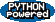EditText of this page (last modified 2011-08-31 21:12:05) FindPage by browsing, title search , text search or an index Or try one of these actions: AttachFile, DeletePage, LikePages, LocalSiteMap, SpellCheck